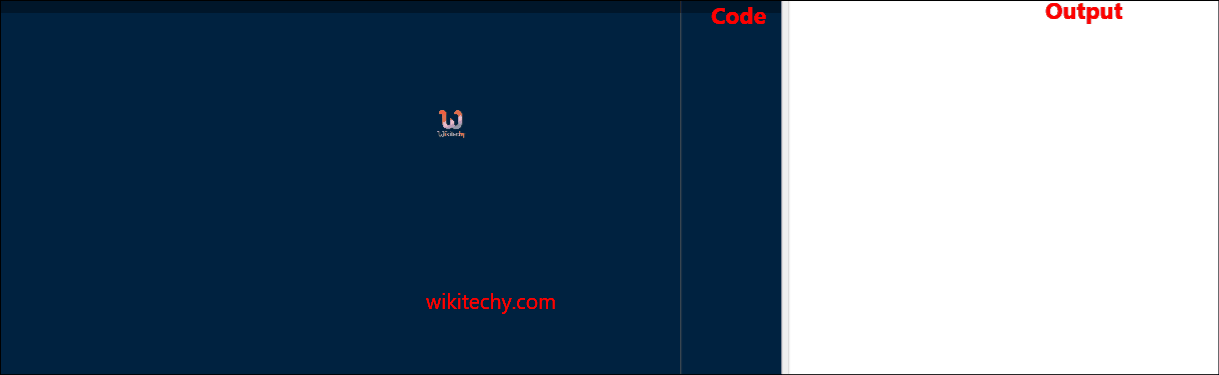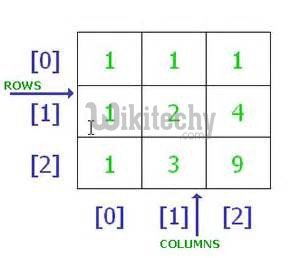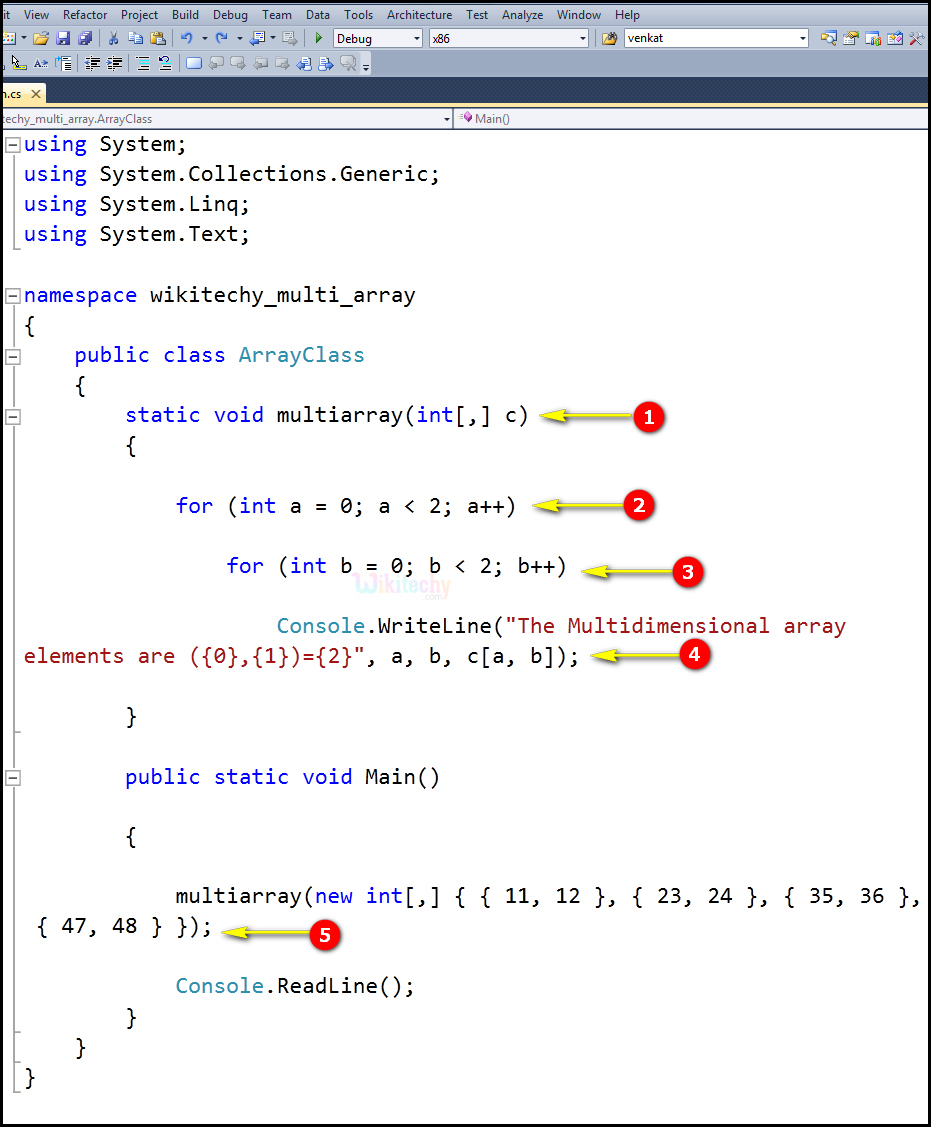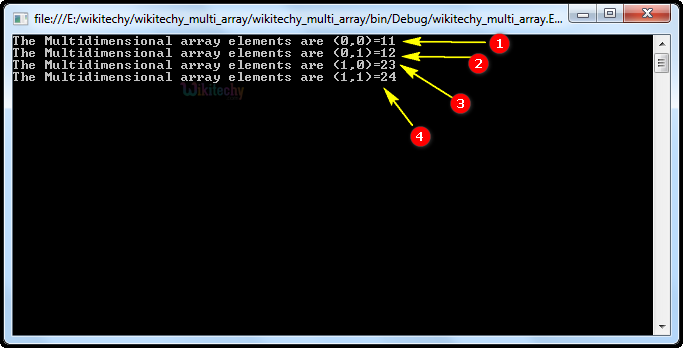# C# multidimensional array | Multidimensional array c# - c# - c# tutorial - c# netC# Multidimensional Array

## What is multidimensional array in C# ?

• The multi-dimensional array in C# is such type of array that contains more than one row to store data on it.
• The multi-dimensional array is also known as rectangulararray in C# because it has same length of each row.
• It can be two dimensional array or three dimensional array or more.
• Multi-dimensional array contains more than one coma (,) within single rectangular brackets ("[ , ]").
• C# supports multidimensional arrays. The simplest form of the multidimensional array is the two-dimensional array.## Code Explanation:1. In this example, a two-dimensional array is initialized and passed to the multarray method, where its elements are displayed.
2. Here for (inta = 0; a<2; a++) specifies integer a = 0, which means the expression, (a<2), is true. Therefore, the statement is executed, and a gets incremented by 1 and becomes 2.
3. for (intb = 0; b< 2; b++) specifies integer b = 0, which means the expression, (b< 2), is true. Therefore, the statement is executed, and b gets incremented by 1 and becomes 2.
4. In Console.WriteLine,the Main method specifies its behavior with the statement "The Multidimensional array elements are".to be displayed on the screen.
5. If we choose to declare an array variable without initialization, we must use the new operator to assign an array to the variable.

## Sample C# examples - Output :1. Here in this output the multidimensional array elements (0,0) is represented as a console statement. The multidimensional array elements(0,0) value as "11"
2. Here in this output the multidimensional array elements (0,1) is represented as a console statement. The multidimensional array elements (0,1) value as "12".
3. In this output the multidimensional array elements (1,0) is represented as a console statement. The multidimensional array elements (1,0)value as "23".
4. In this output the multidimensional array elements (1,0) is represented as a console statement. The multidimensional array elements (1,1) value as "24".